## Heat Transfer Miscellaneous

#### Heat-Transfer

1. A long glass cylinder of inner diameter = 0.03 m and outer diameter = 0.05 m carries hot fluid inside. If the thermal conductivity of glass = 1.05 W/mK, the thermal resistance (K/W) per unit length of the cylinder is

1. Given data:
d1 = 0.03 m

 ∴ r1 d1 = 0.3 = 0.015 m 2 2

d2 = .05 m
∴ r2 = .025 m
 K = 1.05 W mK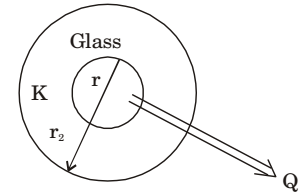Thermal resistance
 Rt = 1 loger22πKL r1

 = 1 loge.0252 × 3.14 × 1.05 × 1 0.015

= 0.077 W/m

##### Correct Option: B

Given data:
d1 = 0.03 m

 ∴ r1 d1 = 0.3 = 0.015 m 2 2

d2 = .05 m
∴ r2 = .025 m
 K = 1.05 W mKThermal resistance
 Rt = 1 loger22πKL r1

 = 1 loge.0252 × 3.14 × 1.05 × 1 0.015

= 0.077 W/m

1. One-dimensional steady state heat conduction takes place through a sol i d whose cross sectional area varies linearly in the direction of heat transfer. Assume t her e is no heat generation in the solid and the thermal conductivity of the material is constant and independent of temperature. The temperature distribution in the solid is

1. We know that one-dimensional steady state heat conduction equation with no heat generation is given by,

 d- kA dT= 0 dx dx

 ⇒ -kA dT = C1 dx

∵ A = Cx + B (linear variation)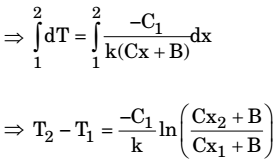Logarithmic temperature distribution

##### Correct Option: C

We know that one-dimensional steady state heat conduction equation with no heat generation is given by,

 d- kA dT= 0 dx dx

 ⇒ -kA dT = C1 dx

∵ A = Cx + B (linear variation)Logarithmic temperature distribution

1. Steady one-dimensional heat conduction takes place across the faces 1 and 3 of a composite slab consisting of slabs A and B in perfect contact as shown in the figure, where kA, kB denote the respective thermal conductivities. Using the data as given in the figure, the interface temperature T2 (in °C) is _________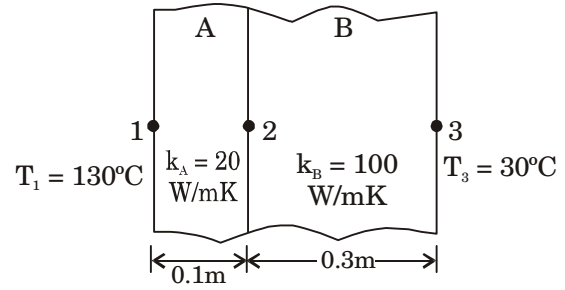1.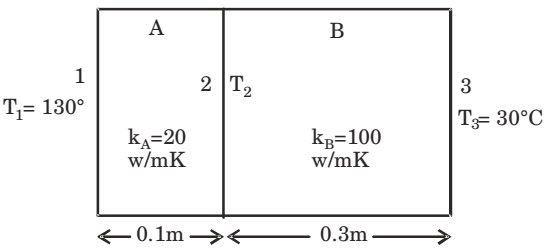Q = kA.(T1 - T2) A LA

 = kB(T2 - T3) LB

 20(130 - T2) = 100(T2 - 30) 0.1 0.3

∴ T2 = 67.5°C

##### Correct Option: AQ = kA.(T1 - T2) A LA

 = kB(T2 - T3) LB

 20(130 - T2) = 100(T2 - 30) 0.1 0.3

∴ T2 = 67.5°C

1. A plastic sleeve of outer radius r0 = 1 mm covers a wire (radius r = 0.5 mm) carrying electric current, Thermal conductivity of the plastic is 0.12 W/mK. The heat transfer coefficient on the outer surface of the sleeve exposed to air is 25 W/m2K. Due to the addition of the plastic cover, the heat transfer from the wire to the ambient will

1.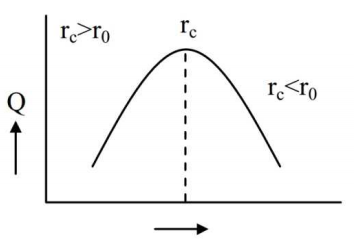r0 = 1 mm, k = 0.15 W/m-k
h = 25 W/m2 -K

 rc = k for cylindrical shape h0

 = 0.15 × 1000 = 0.15 × 40 = 6mm 25

∴ rc > r0
⇒ The heat transfer from the wire to the ambient will increase

##### Correct Option: Ar0 = 1 mm, k = 0.15 W/m-k
h = 25 W/m2 -K

 rc = k for cylindrical shape h0

 = 0.15 × 1000 = 0.15 × 40 = 6mm 25

∴ rc > r0
⇒ The heat transfer from the wire to the ambient will increase

1. A brick wall (k = 0.9 W/mK) of thickness 0.18 m separates the warm air in a room from the cold ambient air. On a particular Winter day, the outside air temperature is - 5°C and the room needs to be maintained at 27°C. The heat transfer coefficient associated with outside air is 20 W/m2K. Neglecting the convective resistance of the air inside the room, the heat loss, in (W/m2), is

1.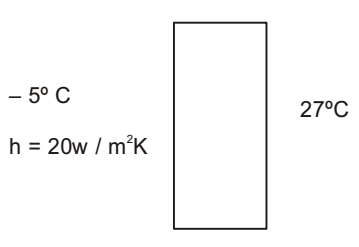Total thermal resistance

 1 = 1 Rth 1 + 1 h k

 1 = 1 = 4 w/m2K Rth 1 + 0.18 20 0.9

 Q = ∆T [27 - (-5)] × 4 = 128 W/m2 Rth

##### Correct Option: CTotal thermal resistance

 1 = 1 Rth 1 + 1 h k

 1 = 1 = 4 w/m2K Rth 1 + 0.18 20 0.9

 Q = ∆T [27 - (-5)] × 4 = 128 W/m2 Rth